Next: The magnetic wheel Up: Alan Turing's Manual for Previous: The B-tube.

Subsections

Miscellaneous special functions

Dummy stops

It is possible by setting certain switches to arrange that the machine stops' when certain instructions are reached. This is effected by arranging that these instructions have no completion signals. The instructions in question are /L and /G. Each of these can be independently made either a time wasting instruction' or a stop.

Dummy stops are very useful in testing routines. The programmer is recommended to insert them at points where major operations may be considered complete. [Note that a /L dummy stop is included in the routine changing sequence'', which brings subroutines into electronic storage from the drum; see p..]

The hooter

When an instruction with function symbol /V is obeyed an impulse is applied to the diaphragm of a loudspeaker. By doing this repeatedly and rhythmically a steady note, rich in harmonies, can be produced. This is used to enable the operator to be called to attend to the machine in some way. The simplest case is where the whole of a job is completed and it is required to clear the electronic stores and start something different. All that is then required is to repeat a cycle of instructions including a hoot, e.g.

FS        NS/V
CS        FS/P
In this case every second instruction will put a pulse into the speaker. These pulses will occur at intervals of 8 beats i.e. 1.92 ms giving a frequency of 521 cycles (about middle C). Or one could use the loop of three instructions
O@          /V
G@        P@/V
M@        G@/P
which gives a slightly louder hoot a fifth lower in frequency. Single pulses applied to the loudspeaker are distinctly audible as something between a tap, a click, and a thump. This fact can be turned to good account. By putting hoot instructions into programmes at suitable points one is enabled to listen in' to the progress of the routine. Some indication of what is going on is given by the rhythm of the clicks that are heard.

The hand switches

One can set up a row of twenty digits on twenty switches. This row H' can affect the behaviour of the machine through instructions with function symbol // or /Z. The former of these will be discussed under magnetic transfers. The latter is used for putting small pieces of information into the machine by hand. [The equations for /Z are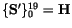,.] Suppose for example that we have a routine for calculating some function of a four character line, and suppose that the calculation takes five minutes. It would then be reasonable to put the arguments in through the switches. This would be particularly so if the arguments used depend partly on the judgment of the experimenter and partly on the values recently obtained, e.g. if one were trying to find a zero of the function, but one was not wishing to repeat the process often enough to mechanise it fully. Again if one were playing chess against the machine this would be the natural way of registering one's moves. [These are allusions to two of Turing's own projects -- his very early work on machine chess, and his project to investigate the zeros of the Riemann zeta function.] (See also the formal mode', pp.-).

The position of the most significant digit

In the calculation of logarithms, reciprocals and square roots it is desirable to be able to standardize' numbers i.e. to express them in the form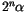where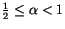. This is made possible at high speed by the use of instructions with the function symbol /@.

[This instruction also has cryptographic applications which Turing does not mention, but which he would have known very well from his work at Bletchley Park, which was then still very secret. Cryptography is also a significant application of the /R function symbol, which counts the number of one bits in a word; Turing refers to this as the sideways adder'' in his quick-reference summary, but does not refer to the instruction at all in the main text of the manual. The relevant equations are:

 Function symbol Equations /R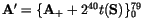/@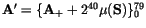where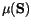is defined as follows:
If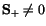, then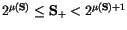If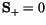, then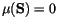and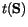is the number of one bits in S.]

The random numbers generator

In the Mark II machine the principle that the state of the machine at one completion signal determines the state at the next is abandoned if instructions with function symbol /W are used. The behaviour of the machine is then to be described as a stochastic' (i.e. chance-controlled) process and is suitable for calculations concerned with stochastic processes. The instruction /W actually puts random digits into the twenty least significant digits of the accumulator.

The following problem is suitable for the use of this facility.

A man in New York starts walking from a street intersection, and at each street intersection decides in which direction to walk by twice tossing a coin (each of the four directions is chosen equally often). It is required to find the probability that before walking twenty blocks he will have succeeded in returning to his starting point. For this purpose New York is to be assumed to be an infinite rectangular lattice of streets and avenues.

The clock

The quantity Z is a twenty digit line which is intended to indicate the time. If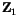and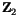are the contents of Z at two times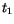and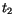, measured in seconds, then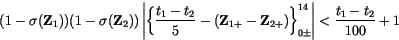This is a way of saying that the first fifteen digits of Z measure the time, the unit being five seconds, to an accuracy of 5%, but that when the most significant digit is 1 the value given cannot be relied on. This only occurs for periods of less than a quarter second at intervals of five seconds.

[Instructions with function symbol /Y set the low 20 digits of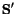to Z.]

The sixty-fifth lines

We mentioned on p.that an extra identification line was added to each magnetic page and that there is a corresponding line on each tube. The only significant effects of these lines are

(a)
They may be seen on the monitor tube with the other lines,

(b)
they are copied from magnetic to electronic store in reading transfers and checked in read-like checking operations (see. p.),

(c)
they can be copied into the accumulator by instructions T@. This is an irregular function, not corresponding to the normal rules. It is intended that it be used with addresses whose first six digits are all 0, i.e. which are names of lines each of which is the first of some page. Under these circumstances the effect of the instruction can be written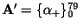where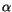is the content of the sixty-fifth line of the page in question. With other addresses the same equation holds, butmust then be interpreted as the content of the short line preceding the line referred to in the address. For example the effect of FET@ is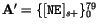. It may be observed that this is the only function connecting a short line with the accumulator.(d)] In loads of odd line-pairs at the end of a page; see p..

[Relative branches]

[Three branch introductions have been introduced so far -- the unconditional /P, the A-conditional /H and the B-conditional /T. These are all absolute branches, setting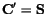if the branch is taken. They have relative variants, /Q, /M and /O respectively, which are identical in their effects except that if the branch is taken, we have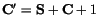.]

The time occupied by various operations

The machine is synchronised by an oscillator with a frequency of 100 kc/s. One cycle (occupying 10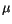s) of this oscillator may be called a digit period'. These digit periods determine the most fundamental rhythm of the machine, but there is another almost equally important rhythm, in which time is divided into beats' of 24 digit periods. Four of these periods form what is called the blackout period' of the beat. The remaining twenty are used for operations on twenty digit rows, each digit being dealt with in one digit period. The prepulses' or completion signals' occur in the blackout periods, and are separated by integral numbers of beats, i.e. by multiples of 240s. As has been mentioned (p.) some instructions overlap their successors, but for programming purposes one may take it that the time consumed in an instruction is that dividing the prepulse initiating it with that initiating its successor. The numbers of beats for each instruction are shown on p.E [in the appendices]. The times for the magnetic instructions are mentioned under the heading of the magnetic wheel.Next: The magnetic wheel Up: Alan Turing's Manual for Previous: The B-tube.
Robert S. Thau 2000-02-13